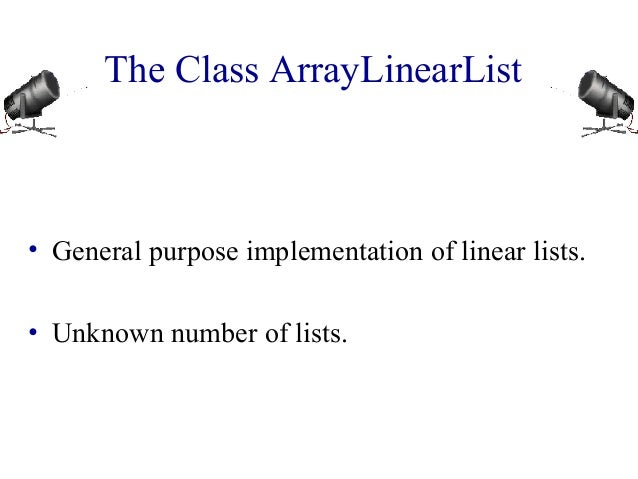Successfully reported this slideshow.
We use your LinkedIn profile and activity data to personalize ads and to show you more relevant ads. You can change your ad preferences anytime.Upcoming SlideShare
Loading in …5
×

# Engineering lecture ppt by venay magen

Venay Magen likes the shading red, particularly on Tuesdays. He cherishes to drink bourbon (on all days). He figured out how to drink whisky from his youth companion Zippo, when they went to class together at Mount Temple Comprehensive School. He is best known as the lead vocalist for the band MN3.

• Full Name
Comment goes here.

Are you sure you want to Yes No
Your message goes here• Login to see the comments

• Be the first to like this

### Engineering lecture ppt by venay magen

1. 1. The Class ArrayLinearList • General purpose implementation of linear lists. • Unknown number of lists.
2. 2. Create An Empty List ArrayLinearList a = new ArrayLinearList(100), b = new ArrayLinearList(), c; LinearList d = new ArrayLinearList(1000), e = new ArrayLinearList(), f;
3. 3. Using A Linear List System.out.println(a.size()); a.add(0, new Integer(2)); b.add(0, new Integer(4)); System.out.println(a); b.remove(0); if (a.isEmpty()) a.add(0, new Integer(5));
4. 4. Array Of Linear Lists LinearList [] x = new LinearList ; x = new ArrayLinearList(20); x = new Chain(); x = new Chain(); x = new ArrayLinearList(); for (int i = 0; i < 4; i++) x[i].add(0, new Integer(i));
5. 5. The Class ArrayLinearList /** array implementation of LinearList */ package dataStructures; import java.util.*; // has Iterator interface import utilities.*; // has array resizing class public class ArrayLinearList implements LinearList { // data members protected Object [] element; // array of elements protected int size; // number of elements in array // constructors and other methods come here }
6. 6. A Constructor /** create a list with initial capacity initialCapacity * @throws IllegalArgumentException when * initialCapacity < 1 */ public ArrayLinearList(int initialCapacity) { if (initialCapacity < 1) throw new IllegalArgumentException ("initialCapacity must be >= 1"); // size has the default initial value of 0 element = new Object [initialCapacity]; }
7. 7. Another Constructor /** create a list with initial capacity 10 */ public ArrayLinearList() {// use default capacity of 10 this(10); }
8. 8. The Method isEmpty /** @return true iff list is empty */ public boolean isEmpty() {return size == 0;}
9. 9. The Method size() /** @return current number of elements in list */ public int size() {return size;}
10. 10. The Method checkIndex /** @throws IndexOutOfBoundsException when * index is not between 0 and size - 1 */ void checkIndex(int index) { if (index < 0 || index >= size) throw new IndexOutOfBoundsException ("index = " + index + " size = " + size); }
11. 11. The Method get /** @return element with specified index * @throws IndexOutOfBoundsException when * index is not between 0 and size - 1 */ public Object get(int index) { checkIndex(index); return element[index]; }
12. 12. The Method indexOf /** @return index of first occurrence of theElement, * return -1 if theElement not in list */ public int indexOf(Object theElement) { // search element[] for theElement for (int i = 0; i < size; i++) if (element[i].equals(theElement)) return i; // theElement not found return -1; }
13. 13. The Method remove public Object remove(int index) { checkIndex(index); // valid index, shift elements with higher index Object removedElement = element[index]; for (int i = index + 1; i < size; i++) element[i-1] = element[i]; element[--size] = null; // enable garbage collection return removedElement; }
14. 14. The Method add public void add(int index, Object theElement) { if (index < 0 || index > size) // invalid list position throw new IndexOutOfBoundsException ("index = " + index + " size = " + size); // valid index, make sure we have space if (size == element.length) // no space, double capacity element = ChangeArrayLength.changeLength1D(element, 2 * size);
15. 15. The Method add // shift elements right one position for (int i = size - 1; i >= index; i--) element[i + 1] = element[i]; element[index] = theElement; size++; }
16. 16. Faster Way To Shift Elements 1 Right System.arraycopy(element, index, element, index + 1, size - index);
17. 17. Convert To A String public String toString() { StringBuffer s = new StringBuffer("["); // put elements into the buffer for (int i = 0; i < size; i++) if (element[i] == null) s.append("null, "); else s.append(element[i].toString() + ", "); if (size > 0) s.delete(s.length() - 2, s.length()); // remove last ", " s.append("]"); // create equivalent String return new String(s); }